The Precision Farming Primer      Appendix D: Case Study in Precision Farming © 1999 Precision Farming Primer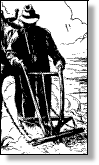Overview of the Case Study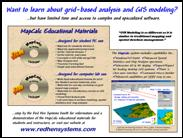Author’s note:  An educational version of the MapCalc software by Red Hen Systems used in the case study provides “hands-on” exercises for the topics discussed in this appendix.   See www.redhensystems.com for more information and ordering; US\$21.95 for Student CD and US\$495.00 for Instructor CD with multi-seat license for a computer lab (prices subject to change).

Now that precision farming is beginning its second decade, where are we?  Yield mapping is gaining commonplace status for many crops and locales.  Site-specific management of field fertilization has a growing number of users.  Remote sensing applications from satellite imagery to aerial photos and on the ground “proximal” sensing are coming onboard.  Irrigation control, field leveling, variable rate seeding, on-farm studies, disease/pest modeling, stress maps and a myriad other computer mapping uses are on the horizon.

Fundamental to all of these applications are the digital nature of a computer map and the procedures required to turn these data into useful information for decision-making.  The bottom line for precision farming appears to be an understanding of the numbers and what one can do with them to enhance their interpretation.

The next several columns will apply map analysis techniques in a case study format.  We will begin with a series of techniques used to visualize and summarize field data and end with several techniques that develop maps to guide management action.  The focus will be on methodology (a.k.a., the precision farming “toolkit”) and not on the scientific results or generalizations about the complex biological, adaphic or physiographic factors driving crop production in the example field.  These intricate relationships are left to agricultural scientists to scrutinize, interpret and put into context with numerous other crops and fields.

The objective of the series of articles simply is to demonstrate the analysis procedures on a consistent data set.  The intent is to build a case study for a single field that illustrates the wealth of emerging analysis capabilities that are available to scientists, agronomists, crop consultants and producers.

One might argue that we have “the technical cart in front of the scientific horse.”  In many instances that appears true.  We can collect data, view the numbers as maps or summary statistics, but struggle to make sense of it all.  In part, the disconnect isn’t technical versus science but the predicament of “the chicken or the egg coming first.”

We have a radically different set of analysis tools than those available just ten years ago.  Concurrently, we have a growing number of farmers collecting geo-registered data, such as crop yield, soil nutrients and meteorological conditions.  The computer industry is turning out software and machines that are increasing more powerful, yet costing less and less and are easier to use.  The ag equipment companies are developing implements and instruments that respond to “on the go” movements throughout a field.  In short, the pieces are coming together.

The accompanying table lists the topics that will be covered in the series.  Most of details of the topics have been discussed in previous “GIS Toolbox” articles.  What awaits is the demonstration of their application to a consistent data set.  Hopefully, the case study will stimulate innovative thinking as much as it outlines how the procedures can be applied.  All of the processing will be done with readily available software for a PC environment.

The next decade in precision farming will focus on making sense of the site-specific relationships we measure.  The technology is here (analysis toolbox) to bridge the gap between maps and decisions.  Its widespread application will be determined by how effective we are in applying it.

# Case Study in Agricultural Map Analysis

## Mapped Data Visualization and Summary

Preprocessing and Map Normalization

Comparing Maps and Temporal Analysis

Interpolating and Assessing Map Surfaces

Integrating Remote Sensing Data

Delineating Management Zones

Clustering Groups of Similar Data

Measuring Correlation Among Maps

Developing Predictive Models

Generating Management Action Maps

Before we start wrestling with the details of spatial data analysis it makes sense to consider some larger issues surrounding their application—namely, the interaction between technology and science, the relevant expression of results, and the current field-centric orientation of analysis.

The underlying concept of precision agriculture is that site-specific actions are better than whole-field ones.  In applying fertilizer, for example, changing the rate and mixture of the nutrients throughout a field puts more than the average where it is needed and less where it is not needed.  However, precise science in the formulation of prescription maps is critical.

To date the infusion of site-specific science has not kept pace with the technologies underlying site-specific agriculture.  We can produce maps of yield variation and a host of potential driving factors, from soil properties and nutrients to electric conductivity and topographic relief.  We can generate prescription maps that guide the precise application of various materials.  Where we used to use one recommendation, we now have several for different locations in a field.  So what’s the problem?

It’s not that there is a problem; it’s just that there is far more potential.   Introduction of spatial technology has forever altered agriculture—for the researcher, as well as the farmer.  The traditional research paradigm involves sampling a very small portion of a field then analyzing these data without reference to their spatial context and relationships.  The most frequently used method develops a “regression model” of one variable on another(s), then extrapolates the results for large geographic regions.

A classic example is a production curve that relates various levels of a nutrient to yield—e.g., the Yield vs. Phosphorous plots you encountered in agronomy 101.  The interpretation of the curve is that if a certain level of phosphorous is present, then a certain amount of yield is expected.  This science, plus a whole lot of field experience (windage), is what goes into a prescription—whole-field and site-specific alike.  If a map of current phosphorous levels exists, then the application rate can be adjusted so that each location receives just the right amount to put it at the right spot on the production curve.

Current precision ag technology enables just that…site-specific application, but based on state-wide equations (science).  In fact, the “rules” used in most systems were developed years ago at experiment stations miles away, indifferent to geographic patterns of topographic, adaphic and biological conditions, and analyzed with non-spatial statistics that intentionally ignore spatial autocorrelation.

Today, an opportunity exists for site-specific science to “tailor” the decision rules for different conditions within a field.  For example, more phosphorous might be applied to south-facing toe slopes than north-facing head-slopes.  But the least amount would be applied in flat or depressed areas.  Of course the spatially-specific rule set would change for different soil types and in accordance with the level of organic matter.

The primary difference between “non-spatial science” and its spatial cousin is the analysis of map variables versus a small set of sample plots attempting to replicate all possible conditions and their extent within a field.  Until recent developments there wasn’t a choice, as the enabling spatial technologies didn’t exist ten years ago.

Now that we have spatial tools we need to infuse them into agricultural science.  Ideally, these activities will gravitate from the experiment station model to extensive on-farm studies that encompass actual conditions on a farmer’s land.  In addition, the focus will expand from identifying “management zones” to the analysis of continuous map surfaces.  Without shifts in perspective, the “whole-field” approach simply will be replaced by “smaller-field-chunks” (management zones) that blindly apply non-spatial rule sets.  Without fully engaged science, the full potential of site-specific agriculture will be aggregated into a series of half steps dictated by technology.

In addition to a closer science/technology marriage, the results of its application need to be expressed in new and more informative ways.  The introduction of maps has enlightened farmers and researchers alike to the variability within agricultural fields.  Interactive queries of a stack of maps identify conditions at specific locations.  However, simply presenting base map data doesn’t always translate into information for decision-making.  Further processing is needed to decode these data into a decision context.  For example, bushels and pounds per acre could be rendered into revenue, cost and net profit maps under specified market conditions.  As the assumptions are changed the economic maps would change similar to a spreadsheet.

Broadening the geographic focus of precision agriculture is a third force directing the future development.  To date, most spatial analysis of farm inputs and outputs stop at the edge of the fields… like the fields are islands surrounded by nothing.  This myopic mindset disregards the larger geographic context of agriculture.  Increasing environmental concern in farm practices could radically alter the field focus in the not too distant future.

For example, the focus might be extended to the surface flows and transport of materials (fine soil particles, organic matter, chemicals, etc.) that connect a field to its surroundings.  Like forestry companies, the cumulative environment effects within an air- or watershed might need to be considered before certain farming activities can take place.  The increasing urban/farm interface will surely alter the need and methods of communication between agriculture and other parts of society.  Spatial analysis can play a key role in how agricultural stewardship is effectively communicated.

Precision agriculture is not going away.  The blend of modern science and technology is like a locomotive with momentum—impossible to stop.  But the direction of the tracks determines where it goes.  Current decisions by software developers, equipment manufacturers, service providers and farmers themselves, are laying the groundwork for tomorrow’s direction.  Thinking “out of the box” at this stage might be the best route.

The next several columns will apply map analysis techniques in a case study format.  Discussion begins with a series of procedures used to visualize and summarize field data and ends with several approaches that develop maps to guide management action.  The map-ematical procedures used to translate data into useful information is demonstrated using a subset of the data collected for a large multidisciplinary project of the Water Management Unit of ARS and the Experiment Station at Colorado State University.  The MapCalc software by Red Hen Systems is used throughout the case study for analysis and display of the data.  A set of “hands-on” exercises based on the case study is available for classroom and self-study (see author’s note).

The most fundamental concept in analyzing precision farming data is the recognition that all of the maps are “numbers first, pictures later.  While the human mind is good at a lot of things, noting subtle differences within large, complex sets of spatial data isn’t one of them.  To visualize these data we first must aggregate the detailed information into a few discrete categories then assign colors to each of the groupings.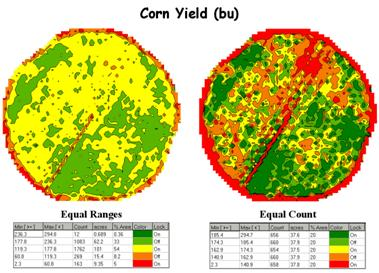Figure 1.  Two different renderings (categorizations) of yield data.

For example, the yield maps shown in figure 1 appear dramatically different but were generated from the same harvest data.  Both maps have five categories extending from low to high yield and use the same color scheme.

The differences in appearance arise from the methods used in determining the breakpoints of the categories—the one on the left uses “Equal Ranges” while the on the right uses “Equal Count.”  Equal Ranges is similar to an elevation contour map since it divides the data range (2.33 to 295 bu/ac) into five steps of about 60 bushels each.  Note that the middle interval (119.3 to 177.8) has more than 100 acres and comprises over half of the field (54%).  Equal Count, on the other hand, divides the same data into five classes, but this time each category represents about the same area (20%) and contains a similar number of samples (about 660).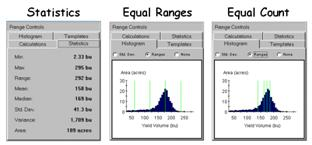Figure 2.  Statistical summary of yield data.

The differences between the two approaches are best visualized from a combined statistical/graphical perspective.  The left panel in figure 2 shows the basic Descriptive Statistics for the data set—min, max, range, mean, median, standard deviation, variance and total area.  While these statistics report a fairly good harvest overall (an average yield of 158 bu/ac), they fail to map the yield patterns (spatial variability) throughout the field.

The two histograms on the right side of figure 2 link the statistical perspective with the map displays.  Recall that a histogram is simply a plot of the data range (X-axes) versus the number of samples (Y-axis).  The “vertical bars” superimposed on the histograms identify the breakpoints for the categories—evenly spaced along the Y-axis for Equal Ranges while variably spaced for Equal Count.  Note the direct relationship between the placement of the bars and the category intervals shown in the map legends shown in figure 1.  For example, the first interval begins at 2.3 bushels for both maps but breaks at 60.8 for Equal Ranges and 140.9 for Equal Count.  The differences in the placement of the breakpoints (vertical bars) account for the differences in the appearance in the two yield maps.

Similarly, changes in the number of intervals, and to a lesser degree, changes in the color scheme will produce different looking maps.  By its very nature, discrete contour mapping aggregates the spatial variability within field-collected data.  The assignment into categories is inherently a subjective process and any changes in the contouring parameters result in different map renderings.  Since decisions are based on the appearance of spatial patterns, different actions could be taken depending on which visualization of the actual data was chosen.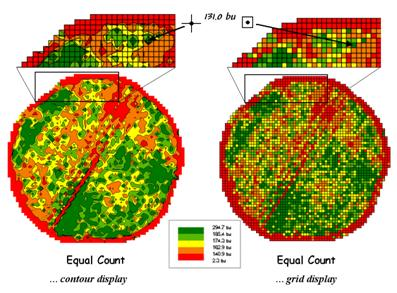Figure 3.  A reference grid is used to directly link the map display and the stored data.

The map on the left-side of figure 3 shows the Equal Count contour map of yield composed of irregular polygons that identify the discrete categories.  The inset directly above the contour map characterizes the underlying lattice data structure for a portion of the field.  A yield value is stored for each of the line intersections of the superimposed regular grid.  The breakpoint values for the map categories are plotted along the lines connecting the actual data.  The contours are formed by connecting the breakpoints, smoothing the boundary lines and filling the polygons with the appropriate color.

The grid data structure utilizes a superimposed reference grid as well.  However, as depicted in the grid map and inset on the right side of figure 3, the centroid of each grid cell is assigned the actual value and the entire cell is filled with the appropriate color.  Note the similarities and differences in the yield patterns between the contour and grid displays—best yields generally in the southeast and northwest portions of the field.

Next month’s column will investigate the important considerations in representing precision farming data as continuous map surfaces, viewing these data as 3-D displays and pre-processing procedures used to “normalize” maps for subsequent analysis.

Preprocessing and normalization are critical steps in map analysis that are often overlooked when the sole objective is to generate graphical output.  In analysis, however, map values and their numerical relationships takes center stage.

Preprocessing involves conversion of raw data into consistent units that accurately represents field conditions.  For example, a yield monitor’s calibration is used to translate electronic signals into measurements of crop production units, such as bushels per acre (measure of volume) or tons per hectare (measure of mass).  The appropriate units (e.g., bushels vs. tons) and measurement system (English acres vs. metric hectares) is determined and applied to the raw data.  While this point is important to software developers, it is somewhat moot in most practical applications after the initial installation of software preferences.

A bit more cantankerous preprocessing concern involves raw data adjustments and corrections.  Like calibrations, adjustments involve “tweaking” of the values… sort of like a slight turn on that bathroom scale to alter the reading to what you know is your true weight.

Corrections, on the other hand, dramatically change measurement values, both numerically and spatially.  For example, as discussed in previous columns the time lag from a combine’s header to the yield monitor can require considerable repositioning of yield measurements.  Also, a combine can pass over the same place more than once and the duplicate records must be corrected.  Even more difficult is the corrections of a reading from a partial header width or the effects of empting and filling the thrashing bin as a combine exits from one pass and enters another.

The old adage “garbage in, garbage out” holds true for any data collection endeavor.  Procedures for calibration, adjustments and corrections are used to translate raw data measurements into the most accurate map values possible.  But even the best data often needs a further refinement before it is ready for analysis.

Normalization involves standardization of a data set, usually for comparison among different types of data.  For example, crop rotation can provide different views of the productivity of a field.  While direct visual comparison of onion and corn yield maps might provide insight, some form of normalization of the data is needed prior to any quantitative analysis.  In a sense, normalization techniques allow you to “compare apples and oranges” using a standard “mixed fruit scale of numbers.”

The most basic normalization procedure uses a goal to adjust map values.  For example, a goal of 250 bushels per acre might be used to normalize a yield map for corn.  The equation,

Norm_GOAL = (mapValue / 250 ) * 100

derives the percentage of the goal achieved by each location in a field.  In evaluating the equation, the computer substitutes a map value for a field location, completes the calculation, stores the result, and then repeats the process for all of the other map locations.

Insert B in figure 1 shows the results of goal normalization.  Note the differences in the descriptive statistics between the original and normalized data— a data range of 2.33 to 295 with an average of 158 bushels per acre for the original data versus .934 to 118 with an average of 63.3 percent of the goal.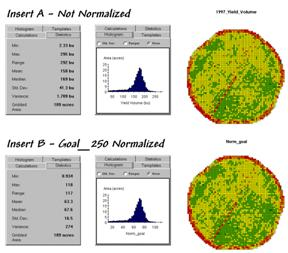Figure 1.  Comparison of original and “goal normalized” data.

Now note that the two histograms look nearly identical and that the same holds for the two maps.  In theory, the histogram and map patterns are identical.  The slight differences in the figure are simply an artifact of rounding the display intervals for the rescaled graphics.  While the descriptive statistics are different, the relationships (patterns) in the normalized histogram and map are identical to the original data.

That’s an important point— both the numeric and spatial relationships in the data are preserved during normalization.  In effect, normalization simply “rescales” the values like changing from one set of units to another (e.g., switching from bushels per acre to cubic meters per hectare).  The significance of the goal normalization is that the new scale allows comparison among different fields and even crop types— the “mixed fruit” expression of apples and oranges.Appendix_D_files\image012.png

Other commonly used normalization expressions are 0-100 and SNV.  0-100 normalization forces a consistent range of values by spatially evaluating the equation

Norm_0-100 = ((mapValue – min) * 100) / (max – min), where max and min are the

maximum and minimum values of the original data.

The result is a rescaling of the data to the range 0 to 100 while retaining the same relative numeric and spatial patterns of the original data.

While goal normalization benchmarks a standard value without regard to the original data, the 0-100 procedure rescales the original data range to a fixed, standard range.  The third normalization procedure, standard normal variable (SNV), uses yet another approach.  It rescales the data based on the central tendency of the data by applying the equation

Norm_SNV = ((mapValue - mean) / stdev) * 100, where mean is the average and stdev

is the standard deviation of the original data.

The result is rescaling of the data to the percent variation from the average.  Mapped data expressed in this form enables you to easily identify “statistically unusual” areas— +100% locates areas that are one standard deviation above the typical yield; -100% locates areas that are one standard deviation below

.

Map preprocessing and normalization are often forgotten steps in a rush to make a map, but they are critical pre-cursor to a host of subsequent analyses.  For precision farming to move beyond pretty maps, the map values themselves become the focus— both their accuracy and their appropriate scaling.

Map normalization is often a forgotten step in the rush to make a map, but is critical to a host of subsequent analyses from visual map comparison to advanced data analysis.  The ability to easily export the data in a universal format is just as critical.  Instead of a “do-it-all” GIS system, data exchange exports the mapped data in a format that is easily consumed and utilized by other software packages.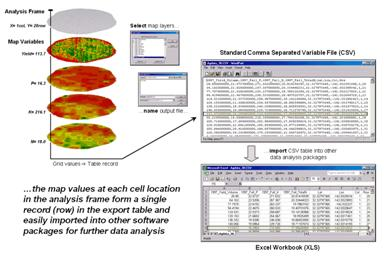Figure 1. The map values at each grid location form a single record in the exported table.

Figure 1 shows the process for grid-based data.  Recall that a consistent analysis frame is used to organize the data into map layers.  The map values at each cell location for selected layers are reformatted into a single record and stored in a standard export table that, in turn, can be imported into other data analysis software.

The example in the figure shows the procedure for exporting a standard comma separated variable (CSV) file with each record containing the selected data for a single grid cell.  The user selects the map layers for export and specifies the name of the output file.  The computer accesses the data and constructs a standard text line with commas separating each data value.  Note that the column, row of the analysis frame and its latitude, longitude earth poison is contained in each record.  In the example, the export file is brought into Excel for further processing.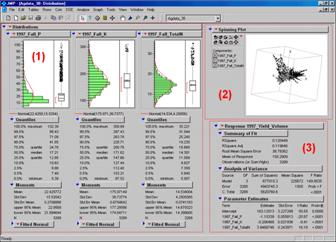Figure 2.  Mapped data can be imported into standard statistical packages for further analysis.

Figure 2 shows the agricultural data imported into the JMP statistical package (by SAS).  Area (1) shows the histograms and descriptive statistics for the P, K and N map layers shown in figure 2.  Area (2) is a “spinning 3D plot” of the data that you can rotate to graphically visualize relationships among the map layers.  Area (3) shows the results of applying a multiple linear regression model to predict crop yield from the soil nutrient maps.  These are but a few of the tools beyond mapping that are available through data exchange between GIS and traditional spreadsheet, database and statistical packages—a perspective that integrates maps with other technologies.

Modern statistical packages like JMP “aren’t your father’s” stat experience and are fully interactive with point-n-click graphical interfaces and wizards to guide appropriate analyses.  The analytical tools, tables and displays provide a whole new view of traditional mapped data.  While a map picture might be worth a thousand words, a gigabyte or so of digital map data is a whole revelation and foothold for site-specific decisions.

One of the most fundamental operations in map analysis is the comparison of two maps.  Questions like “how different are the maps?”, “how are they different?” and “where are they different?” immediately spring to mind.  Quantitative answers are needed because visual comparison cannot fully consider all of the detail in an objective manner.

Recall that there are two basic forms of mapped data used in precision farming— discrete maps (vector) and map surfaces (grid).  Let’s consider discrete map comparison first.  The two maps shown in figure 1 identify corn yield for successive seasons on a central-pivot field in Colorado.  Note that the maps have been normalized to 300 bu/ac and displayed with a common color pallet… but how different are they; how are they different; and where are they different?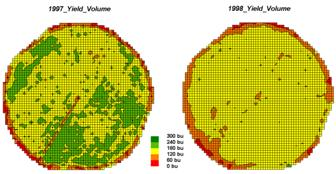Figure 1.  Discrete Yield Maps for Consecutive Years.

While your eyes flit back and forth in an attempt to compare the maps, the computer approaches the problem more methodically.  The first step converts the vector contour lines to a grid value for each cell.  An analysis grid resolution is chosen (50ft cells are used in this example) and geometrically aligned with the maps.  The dominant yield class within each cell is assigned its interval value (values 1 through 5 corresponding to the color range).  The grid mesh used is superimposed on the yield maps for visual reference.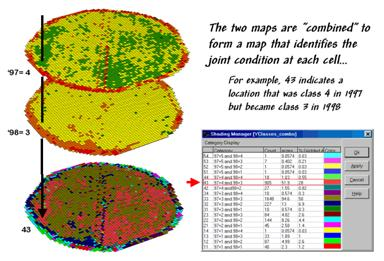Figure 2.  Coincidence Map Identifying the Conditions for Both Years.

The next step, as shown in figure 2, combines the two maps into a single map that indicates the “joint condition” for both years.  Since the two maps have identical gridding, the computer simply retrieves the two class assignments for a grid location then converts them to a single number.

The map-ematical procedure merely computes the “first value times ten plus the second value” to form a compound number.  In the example shown in the figure, the value “forty-three” is interpreted as class 4 in the first year but decreasing to class 3 in the next year.

The final step sums up the changes to generate a coincidence table (see figure 3).  The columns and rows in the table represent the class assignments on the 1997 and 1998 yield maps, respectively.  The body of the table reports the number of cells for each joint condition.  For example, column 4 and row 3 notes that there are 905 occurrences where the yield class slipped from level four (180-240bu/ac) to level three (120-180bu/ac).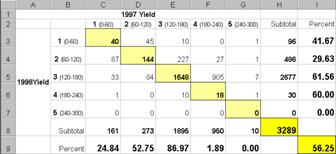Figure 3.  Coincidence Summary Table.

The off-diagonal entries indicate changes between the two maps—the values indicate the relative importance of the change.  For example, the 905 statistic for the “four-three” change is the largest and therefore identifies the most significant change in the field.  The 0 statistic for the “four-one” combination indicates that level four never slipped clear to level 1.

The diagonal entries summarize the agreement between the two maps.  The greatest portion of the field that didn’t change occurs for yield class 3 (“three-three” with 1640 cells).  The statistic in the extreme lower-right (56.25%) reports that only a little more than half the field didn’t change its yield class.

Generally speaking, the maps are very different (only 56.25% unchanged).  The greatest difference occurred for class 4 (only 1.89% didn’t change).  And a detailed picture of the spatial patterns of change is depicted in the coincidence map.  That’s a lot more meat in the answers to the basic map comparison questions (how much, how and where) than visceral viewing can do.  Next time we’ll look at even more precise procedures for reporting the differences.

Contour maps are the most frequently used and familiar form of presenting precision ag data.  The color-coded ranges of yield (0-60, 60-120, …etc. bushels) shown on the floor of the 3-D plots in figure 1 are identical to the discrete maps discussed in last month’s column.  The color-coding of the contours is draped for cross-reference onto the continuous 3-D surface of the actual data.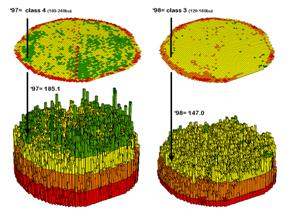Figure 1.  3-D Views of Yield Surfaces for Consecutive Years.

Note the “spikes and pits” in the surfaces that graphically portray the variance in yield data for each of the contour intervals.  While discrete map comparison identifies shifts in yield classes, continuous surface comparison identifies the precise difference at each location.

For example, a yield value of 179 bushels and another one of 121 for successive years are both assigned to the third contour interval (120 to180; yellow).  A discrete map comparison would suggest that no change in yield occurred for the location because the contour interval remained constant.  A continuous surface comparison, on the other hand would report a fairly significant 58-bushel decline.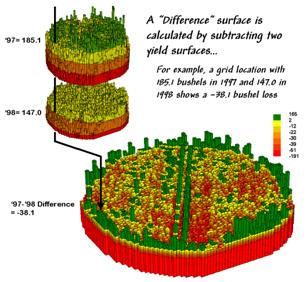Figure 2.  A Difference Surface Identifies the Change in Yield.

Figure 2 shows the calculations using the actual values for the same location highlighted in the previous month’s discussion.  The discrete map comparison reported a decline from yield level 4 (180 to 240) to level 3 (120 to 180).

The continuous surface comparison more precisely reports the change as –38.1 bushels.  The differences for other 3,287 grid cells are computed to derive the Difference Surface that tracks the subtle variations in the spatial pattern of the changes in yield.

The MapCalc command, “Compute Yield_98 minus Yield_97 for Difference” generates the difference surface.  If the simple “map algebra” equation is expanded to “Compute (((Yield_98 minus Yield_97) / Yield_97) *100)” a percent difference surface would be generated.  Keep in mind that a map surface is merely a spatially organized set of numbers that awaits detailed analysis then transformation to generalized displays/summaries for human consumption.

In figure 2, note that the wildest differences (green spikes and red pits) occur at the field edges and along the access road—from an increase of 165 bushels to a decrease of 191 bushels.  However, notice that most of the change is about a 25-bushel decline (mean= -22.6; median= -26.3) as denoted in figure 3.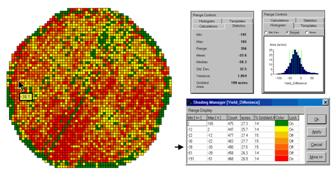Figure 3.  A 2-D Map and Statistics Describe the Differences in Yield.

The histogram of the yield differences shows the numerical distribution of the data.  Observe that it is normally distributed and that the bulk of the data is centered about a 25-bushel decline.  The vertical lines in the histogram locate the contour intervals used in the 2-D display that shows the geographic distribution of the data.

The detailed legend links the color-coding of the map intervals to some basic frequency statistics.  The example location with the calculated decline of –38.1 is assigned to the –39 to –30 contour and displayed as a mid-range red tone.  The display uses an Equal Count method with seven intervals, each representing approximately 15% of the field.  Green is locked for the only interval of increased yield.  The decreased yield intervals form a color-gradient from yellow to red.  All in all, much more information in a more effective form than simply taping a couple of yield maps to a wall for visual comparison.

The ability to map-ematically evaluate continuous surfaces is fundamental to precision farming.  Difference surfaces are one of the simplest and most intuitive forms.  While the math and stat of other procedures are fairly basic, the initial thought of “you can’t do that to a map” is usually a reflection of our non-spatial statistics and paper-map legacies.  In most instances, precision ag processing is simply an extension of current practice from sample plots to mapped data.  The remainder of this case study investigates these extensions.

Soil sampling has long been at the core of agricultural research and practice.  Traditionally point-sampled data were analyzed by non-spatial statistics to identify the “typical” level of a nutrient throughout an entire field.  Considerable effort was expended to determine the best single estimate and assess just how good it was in typifying a field.

However none of the non-spatial techniques make use of the geographic patterns inherent in the data to refine the estimate—the typical level is assumed every the same within a field.  The computed variance (or standard deviation) indicates just how good this assumption is—the larger the standard deviation the less valid is the assumption “everywhere the same.”

Surface modeling, on the other hand, utilizes the spatial patterns in a data to generate localized estimates throughout a field.  Conceptually it “maps the variance” by using geographic position to help explain the differences in sample values.  In practice it simply fits a continuous surface (kind of like a blanket) to the point data spikes.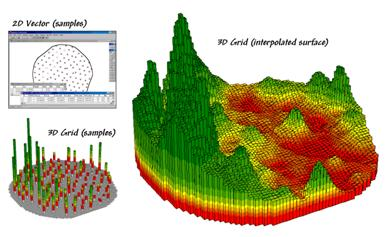Figure 1.  Spatial interpolation involves fitting a continuous surface to sample points.

While the extension from non-spatial to spatial statistics is quite a theoretical leap, the practical steps are relatively easy.  The left side of figure 1 shows a point map of the phosphorous soil samples.  This highlights the principle difference from traditional soil sampling—each sample must be geo-referenced as it is collected.  In addition, the sampling pattern and intensity are often different to maximize spatial information within the collected data (but that story is reserved for another time).

The surface map on the right side of figure 1 depicts the continuous spatial distribution derived from the point data.  Note that the high spikes in the western portion of the field and the relatively low measurements in the center are translated into the peaks and valleys of the surface map.

The traditional, non-spatial approach, if mapped, would be a flat plane (average phosphorous level) aligned within the bright yellow zone.  Its “everywhere the same” assumption fails to recognize the patterns of larger levels (greens) and smaller levels (reds).  A fertilization plan for phosphorous based on the average level (22ppm) would be ideal for the yellow zone but likely inappropriate for a lot of the field as the data vary from 5 to 102ppm.

It’s common sense that nutrient levels vary throughout a field.  However, there are four important considerations before surface modeling is appropriate—

·         the pattern needs to be significant enough to warrant variable-rate application (cost effective),

·         the prescription methods must be spatially appropriate for the pattern (decision rules),

·         the derived map surface must fully reflect a real spatial pattern (map accuracy), and

·         the procedure for generating the pattern must be straightforward and easy to use (usability).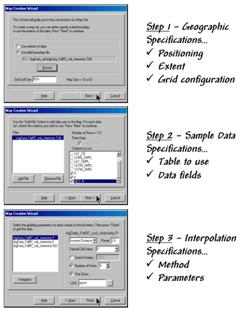Figure 2.  A Wizard interface guides a user through the necessary steps for interpolating sample data.

Let’s tackle the last point on usability first.  The mechanics of generating an interpolated surface involves three steps to specify the geographic, data and interpolation considerations (see figure 2).  The first step establishes the geographic position, field extent and grid configuration to be used.  While these specifications can be made directly, it is easiest to simply reference an exiting map of the field boundary (positioning and extent—AgData_Fall97_soil_chemistry.TAB in this case) then enter the grid spacing to be used (configuration—50 feet).

The next step identifies the table and data fields to be used.  The user navigates to the file (data table— AgData_Fall97_soil_chemistry.TAB) then simply checks the maps to be interpolated (data fields—P, K, NO3_N).

The final step establishes the interpolation method and necessary factors.  In the example, the default Inverse Distance Squared (1/d2) method was employed using the six nearest sample points.  Other methods, such as Krigging, could be specified and would result in somewhat different surfaces.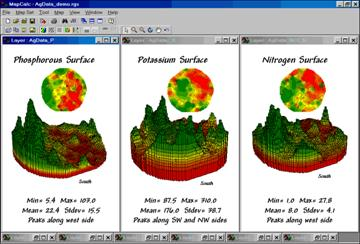Figure 3.  Interpolated Phosphorous, Potassium and Nitrogen surfaces.

Figure 3 shows the P, K, NO3_N map surfaces that were generated by the Interpolation Wizard specifications shown in figure 2.  The entire process including entering specifications, calculation of the surfaces and display took less than a minute.

In each instance the yellow zone contains the average for the entire field.  Visually comparing the relative amounts and patterns of the green and red areas gives you an idea of the difference between the assumption that the average is everywhere and amount of spatial information inherent in the soil sample data.  Next time we’ll investigate how to evaluate the accuracy of these maps—whether they’re good maps or bad maps.

For some, the previous discussion on generating maps from soil samples might have been too simplistic—enter a few things then click on a data file and, viola, you have a soil nutrient surface artfully displayed in 3D with a bunch of cool colors draped all over it.

Actually, it is that easy to create one.  The harder part is figuring out if it makes sense and whether it is something you ought to use in analysis.  The next few columns will investigate how you can tell if the derived map is “a good map, or a bad map.”  This month we’ll assess the relative amounts of spatial information provided in a whole-field average and two different interpolated map surfaces.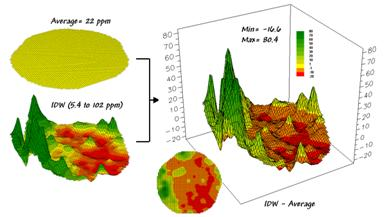Figure 1.  Spatial Comparison of a Whole-Field Average and an IDW Interpolated Map.

The top-left item in Figure 1 shows the map of a field’s average phosphorous levels.  It’s not very exciting and looks like a pancake but that’s because there isn’t any information about spatial variability in an average value—assumes 22ppm is everywhere in the field.

The non-spatial estimate simply adds up all of the sample measurements and divides by the number of samples to get 22ppm.  Since the procedure didn’t consider where the different samples were taken, it can’t map the variations in the measurements.  It thinks the average is everywhere, plus or minus the standard deviation.  But there is no spatial guidance where phosphorous levels might be more, or where they might be less.

The spatially based estimates are shown in the map surface just below the pancake.  As described in the last column, spatial interpolation looks at the relative positioning of the soil samples as well as their measure phosphorous levels.  In this instance the big bumps were influenced by high measurements in that vicinity while the low areas responded to surrounding low measurements.

The map surface in the right portion of figure 1 objectively compares the two maps simply by subtracting them.  The colors were chosen to emphasize the differences between the whole-field average estimates and the interpolated ones.  The yellow band indicates the average level while the progression of green tones locates areas where the interpolated map thought there was more phosphorous.  The progression of red tones identifies the opposite with the average estimate being more than the interpolated ones.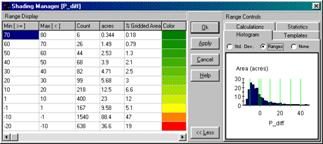Figure 2.  Statistics summarizing the difference between the maps in figure 1.

The information in figure 2 shows that the difference between the two maps ranges from –20 to +80ppm.  If one assumes that +/- 10ppm won’t significantly change a fertilization recommendation, then about two-thirds (47+5.1+12= 64.1 percent of the field) is adequately covered by the whole-field average.  But that leaves about a third of the field that is receiving too much (6.6+3+2.5+2.1+1.3+.79+.18= 16.5 percent) or too little (19 percent) phosphorous under a fertilization program based on the average.

Now turn your attention to figure 3 that compares maps derived by two different interpolation techniques—IDW (inverse distance-weighted) and Krig.  Note the similarity in the peaks and valleys of the two surfaces.  While subtle differences are visible the general trends in the spatial distribution of the data are identical.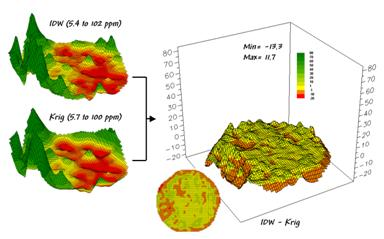Figure 3.  Spatial Comparison of IDW and Krig Interpolated Maps.

The difference map on the right confirms the coincident trends.  The broad band of yellow identifies areas that are +/- 1 ppm.  The brown color identifies areas that are within 10 ppm with the IDW surface estimates a bit more than the Krig ones.  Applying the same assumption about +/- 10 ppm difference being negligible in a fertilization program the maps are effectively identical.

So what’s the bottom line?  That there are substantial differences between a whole field average and interpolated surfaces—at least for this data set.  It suggests that quibbling about the best interpolation technique isn’t as important as using an interpolated surface (any surface) over the whole field average.  However, what needs to be addressed is whether an interpolated surface (any surface) actually reflects the real spatial distribution.  That weighty question awaits the next couple of columns.

Last month’s discussion compared the assumption of the field average with map surfaces generated by two different interpolation techniques for phosphorous levels throughout a field.  While there was considerable differences between the average and the derived surfaces (from -20 to +80ppm), there was relatively little difference between the two surfaces (+/- 10ppm).

But which surface best characterizes the spatial distribution of the sampled data?  The answer to this question lies in Residual Analysis—a technique that investigates the differences between estimated and measured values throughout a field.  It’s common sense that one shouldn’t simply accept a soil nutrient map without checking out its accuracy.  Cool graphics just isn’t enough.

Ideally, one designs an appropriate sampling pattern and then randomly locates a number of “test points” to assess interpolation performance.  Since a lot is riding on the accuracy of the interpolated maps, it makes sense to invest a little bit more just to see how well things are going.

But alas, that wasn’t the case in the AgData data base… nor is it in most cases.  One approach is to randomly remove a small portion of the samples that will be withheld during interpolation, then used to evaluate interpolated estimates.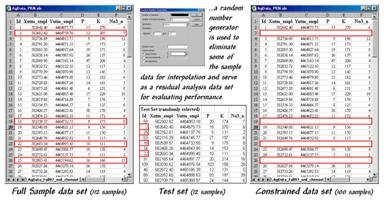Figure 1.  Identifying a test set of samples.

Figure 1 outlines a technique that uses Excel’s “random number generator” to select a test set of samples.  One specifies a number range (1 to 112 for sample IDs), the number of samples desired (12 for a 10% sample) and the distribution for selecting numbers (“uniform” for an equal chance of being selected).  The table in the middle of the figure identifies the twelve randomly selected sample points that were eliminated from the interpolation data set and used to form the test set.

The constrained set data was used to generate two map surfaces of phosphorous.  The map on the left in figure 2 used the default specifications for Inverse Distance Weighted (IDW), while the one on the right used Kriging defaults.  A common color palette from red (0-10ppm) through green (100-110ppm) was applied to both.  The yellow inflection zone encompasses the whole-field average phosphorous level.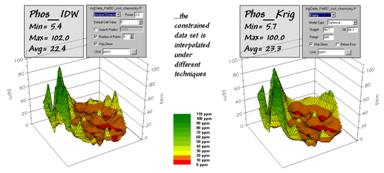Figure 2.  Interpolated IDW and Krig surfaces based on the constrained data set.

Both maps display a similar shape but there are some subtle differences.  The Krig surface appears generally smoother and the IDW surface has four distinct peaks in the northeast portion of the field (right side).  Otherwise the surfaces seem fairly similar as they were for the full set of data discussed last month.

But which surface did a better job at estimating the measured phosphorous levels in the test set?  The table in figure 3 reports the results.  The first column identifies the sample ID and the second column the actual measured value for that location.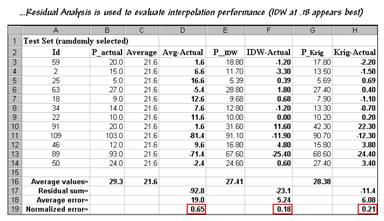Figure 3.  Residual Analysis table assessing interpolation performance.

Column C simply depicts estimating the whole-field average (21.6) at each of the test locations.  Column D computes the difference of the “estimate minus actual”—formally termed the residual.  For example, the first test point (ID#59) estimated average of 21.6 but was measured as 20.0 so the residual is 1.6 (21.6-20.0= 1.6ppm)… not bad.  However, test point #109 is way off (21.6-103.0= -81.4ppm)… nearly 400% error!

The residuals for the IDW and Krig maps are similarly calculated to form columns R and H, respectively.  First note that the residuals for the whole-field average are generally larger than either those for the IDW or Krig estimates.  Next note that the residual patterns between the IDW and Krig are very similar—when one is way off, so is the other and usually by about the same amount.  A notable exception is for test point #91 where Krig dramatically over-estimates.

The rows at the bottom of the table summarize the residual analysis results.  The “Residual sum” row characterizes any bias in the estimates—a negative value indicates a tendency to underestimate with the magnitude of the value indicating how much.  The –92.8 value for the whole-field average indicates a relatively strong bias to underestimate.

The “Average error” row reports how far off on the average each estimate was.  The 19.0ppm figure is three times worse than Krig’s estimated error (6.08) and nearly four times worse than IDW’s (5.24).  Comparing the figures to the assumption that a +/-10ppm is negligible in a fertilization program it is readily apparent that the whole-field estimate is inappropriate to use and that the accuracy differences between IDW and Krig are minor.

The “Normalized error” row simply calculates the average error as a proportion of the average value for the test set of samples (5.24/29.3= .18 for IDW).  This index is the most useful as it allows you to compare the relative map accuracies between different maps.  Generally speaking, maps with normalized errors of more than .10 are very suspect and one might not want to make important decisions using them.

So what’s the bottom line?  Residual Analysis is an important component of precision agriculture.  Without an understanding of the relative accuracy and interpolation error of the base maps, one can’t be sure of the recommendations derived from the data.  The investment in a few extra  sampling points for testing and residual analysis of these data provides a sound foundation for site-specific management.  Without it, the process becomes one of blind faith.

How often have you seen a precision ag presenter “lasso” a portion of a map with a laser pointer and boldly state “See how similar this area is to the locations over here and here” as the pointer rapidly moves about the map.  More often than not, there is a series of side-by-side maps serving as the background scenery for the laser show.

But just how similar is one field location to another?  Really similar, or just a little similar?  And just how dissimilar are all of the other areas?  While visceral analysis can identify broad relationships it takes a quantitative map analysis approach to handle the detailed scrutiny demanded by site-specific management.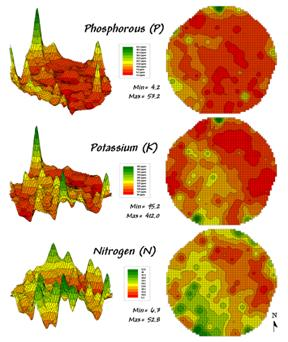Figure 1.  Map surfaces identifying the spatial distribution of P,K and N throughout a field.

Consider the three maps shown in figure 1— what areas identify similar patterns?  If you focus your attention on a location in the southeastern portion how similar are all of the other locations?

The answer to these questions are much too complex for visual analysis and certainly beyond the geo-query and display procedures of standard desktop mapping packages.  The data in the example shows the relative amounts of phosphorous, potassium and nitrogen throughout a central pivot corn field in Colorado.

In visual analysis you move your focus among the maps to summarize the color assignments at different locations.  The difficulty in this approach is two-fold— remembering the color patterns and calculating the difference.  Quantitative map analysis does the same thing except it uses map values in place of colors.  In addition, the computer doesn’t tire as easily as you and completes the comparison for all of the locations throughout the map window (3289 grid cells in this example) in a couple seconds.

The upper-left portion of figure 2 illustrates capturing the data patterns of two locations for comparison.  The “data spear” at map location 45column, 18row identifies the P-level as 11.0, the K-level as 177.0 and N-level as 32.9ppm.  This step is analogous to your eye noting a color pattern of burnt-red, dark-orange and light-green.  The other location for comparison (32c, 62r) has a data pattern of P= 53.2, K= 412.0 and N= 27.9.  Or as your eye sees it, a color pattern of dark-green, dark-green and yellow.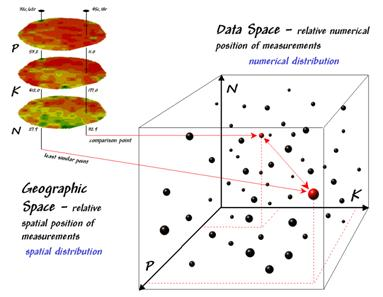Figure 2.  Conceptually linking geographic space and data space.

The right side of figure 2 conceptually depicts how the computer calculates a similarity value for the two response patterns.  The realization that mapped data can be expressed in both geographic space and data space is key to understanding quantitative analysis similarity.

Geographic space uses coordinates, such latitude and longitude, to locate things in the real world—such as the southeast and extreme north points identified in the example field.  The geographic expression of the complete set of measurements depicts their spatial distribution in familiar map form.

Data space, on the other hand, is a bit less familiar.  While you can’t stroll through data space you can conceptualize it as a box with a bunch of balls floating within it.  In the example, the three axes defining the extent of the box correspond to the P, K and N levels measured in the field.  The floating balls represent grid locations defining the geographic space—one for each grid cell.  The coordinates locating the floating balls extend from the data axes—11.0, 177.0 and 32.9 for the comparison point.  The other point has considerably higher values in P and K with slightly lower N (values 53.2, 412.0, 27.9 respectively) so it plots at a different location in data space.

The bottom line for data space analysis is that the position of a point identifies its numerical pattern—low, low, low in the back-left corner, while high, high, high is in the upper-right corner of the box.  Points that plot in data space close to each other are similar; those that plot farther away are less similar.

In the example, the floating ball closest to you is least similar (greatest distance) from the comparison point.  This distance becomes the reference for “most different” and sets the bottom value of the similarity scale (0% similar).  A point with an identical data pattern plots at exactly the same position in data space resulting in a data distance of 0 that equates to the highest similarity value (100% similar).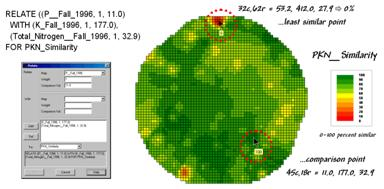Figure 3.  A similarity map identifying how related locations are to a given point.

The similarity map shown in figure 3 applies the similarity scale to the data distances calculated between the comparison point and all of the other points in data space.  The green tones indicate field locations with fairly similar P, K and N levels to the comparison location in the field—with the darkest green identifying locations with identical P, K and N levels.  The red tones indicate increasing dissimilar areas.  It is interesting to note that most of the very similar locations are in the western portion of the field.

A similarity map can be an invaluable tool for investigating spatial patterns in any complex set of mapped data.  While humans are unable to conceptualize more than three variables (the data space box), a similarity index can handle any number of input maps.  Also, the different layers can be weighted to reflect relative importance in determining overall similarity.

In effect, a similarity map replaces a lot of laser-pointer waving and subjective suggestions of similar/dissimilar areas with a concrete, quantitative measurement at each map location.  The technique moves map analysis well beyond the old “I’d never have seen, it if I hadn’t believe it” mode of spatial interpretation.

Last section introduced the concept of “data distance” as a means to measure similarity within a map.  One simply mouse-clicks a location and all of the other locations are assigned a similarity value from 0 (zero percent similar) to 100 (identical) based on a set of specified maps.  The statistic replaces difficult visual interpretation of map displays with an exact quantitative measure at each location.

An extension to the technique allows you to circle an area then compute similarity based on the typical data pattern within the delineated area.  In this instance, the computer calculates the average value within the area for each map layer to establish the comparison data pattern, then determines the normalized data distance for each map location.  The result is a map showing how similar things are to the area of interest.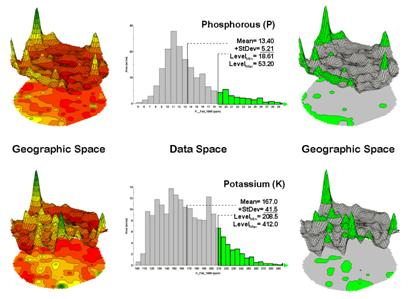Figure 1.  Identifying areas of unusually high measurements.

The link between Geographic Space and Data Space is key.  As shown in figure 1, spatial data can be viewed as a map or a histogram.  While a map shows us “where is what,” a histogram summarizes “how often” measurements occur (regardless where they occur).

The top-left portion of the figure shows a 2D/3D map display of the relative amount of phosphorous (P) throughout a farmer’s field.  Note the spikes of high measurements along the edge of the field, with a particularly big spike in the north portion.

The histogram to the right of the map view forms a different perspective of the data.  Rather than positioning the measurements in geographic space it summarizes their relative frequency of occurrence in data space.  The X-axis of the graph corresponds to the Z-axis of the map—amount of phosphorous.  In this case, the spikes in the graph indicate measurements that occur more frequently.  Note the high occurrence of phosphorous around 11ppm.

Now to put the geographic-data space link to use.  The shaded area in the histogram view identifies measurements that are unusually high—more than one standard deviation above the mean.  This statistical cutoff is used to isolate locations of high measurements as shown in the map on the right.  The procedure is repeated for the potassium (K) map surface to identify its locations of unusually high measurements.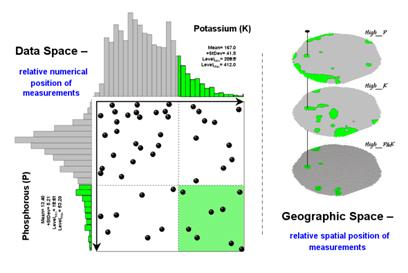Figure 2.  Identifying joint coincidence in both data and geographic space.

Figure 2 illustrates combining the P and K data to locate areas in the field that have high measurements in both.  The graphic on the left is termed a scatter diagram or plot.  It graphically summarizes the joint occurrence of both sets of mapped data.

Each ball in the scatter plot schematically represents a location in the field.  Its position in the plot identifies the P and K measurements at that location.  The balls plotting in the shaded area of the diagram identify field locations that have both high P and high K.  The upper-left partition identifies joint conditions in which neither P nor K are high.  The off-diagonal partitions in the scatter plot identify locations that are high in one but not the other.

The aligned maps on the right show the geographic solution for areas that are high in both of the soil nutrients.  A simple map-ematical way to generate the solution is to assign 1 to all locations of high measurements in the P and K map layers (bight green).  Zero is assigned to locations that aren’t high (light gray).  When the two binary maps (0/1) are multiplied, a zero on either map computes to zero.  Locations that are high on both maps equate to 1 (1*1 = 1).  In effect, this “level-slice” technique maps any data pattern you specify… just assign 1 to the data interval of interest for each map variable.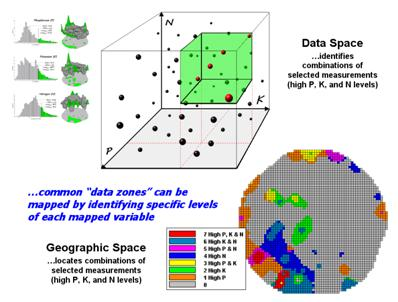Figure 3.  Level-slice classification using three map variables.

Figure 3 depicts level slicing for areas that are unusually high in P, K and N (nitrogen).  In this instance the data pattern coincidence is a box in 3-dimensional scatter plot space.

However a map-ematical trick was employed to get the map solution shown in the figure.  On the individual maps, high areas were set to P=1, K= 2 and N=4, then the maps were added together.

The result is a range of coincidence values from zero (0+0+0= 0; gray= no high areas) to seven (1+2+4= 7; red= high P, high K, high N).  The map values in between identify the map layers having high measurements.  For example, the yellow areas with the value 3 have high P and K but not N (1+2+0= 3).  If four or more maps are combined, the areas of interest are assigned increasing binary progression values (…8, 16, 32, etc)—the sum will always uniquely identify the combinations.

While level-slicing isn’t a very sophisticated classifier, it does illustrate the useful link between data space and geographic space.  This fundamental concept forms the basis for most geostatistical analysis… including map clustering and regression to be tackled in the next couple of sections.

The last couple of topics have focused on analyzing data similarities within a stack of maps.   The first technique, termed Map Similarity, generates a map showing how similar all other areas are to a selected location.  A user simply clicks on an area and all other map locations are assigned a value from 0 (0% similar—as different as you can get) to 100 (100% similar—exactly the same data pattern).

The other technique, Level Slicing, enables a user to specify a data range of interest for each map in the stack then generates a map identifying the locations meeting the criteria.  Level Slice output identifies combinations of the criteria met—from only one criterion (and which one it is), to those locations where all of the criteria are met.

While both of these techniques are useful in examining spatial relationships, they require the user to specify data analysis parameters.  But what if you don’t know what Level Slice intervals to use or which locations in the field warrant Map Similarity investigation?  Can the computer on its own identify groups of similar data?  How would such a classification work?  How well would it work?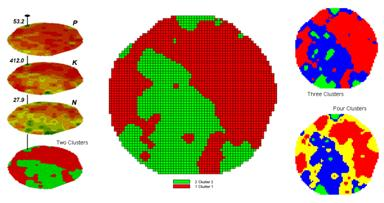Figure 1.  Examples of Map Clustering.

Figure 1 shows some examples derived from Map Clustering.  The “floating” maps on the left show the input map stack used for the cluster analysis.  The maps are the same P, K, and N maps identifying phosphorous, potassium and nitrogen levels throughout a cornfield that were used for the examples in the previous topics.

The map in the center of the figure shows the results of classifying the P, K and N map stack into two clusters.  The data pattern for each cell location is used to partition the field into two groups that are 1) as different as possible between groups and 2) as similar as possible within a group.  If all went well, any other division of the field into two groups would be not as good at balancing the two criteria.

The two smaller maps at the right show the division of the data set into three and four clusters.  In all three of the cluster maps red is assigned to the cluster with relatively low responses and green to the one with relatively high responses.   Note the encroachment on these marginal groups by the added clusters that are formed by data patterns at the boundaries.

The mechanics of generating cluster maps are quite simple.  Simply specify the input maps and the number of clusters you want then miraculously a map appears with discrete data groupings.  So how is this miracle performed?  What happens inside cluster’s black box?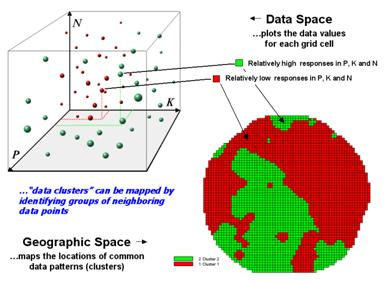Figure 2.  Data patterns for map locations are depicted as floating balls in data space.

The schematic in figure 2 depicts the process.  The floating balls identify the data patterns for each map location (geographic space) plotted against the P, K and N axes (data space).  For example, the large ball appearing closest to you depicts a location with high values on all three input maps.  The tiny ball in the opposite corner (near the plot origin) depicts a map location with small map values.  It seems sensible that these two extreme responses would belong to different data groupings.

The specific algorithm used in clustering was discussed in a previous topic (see “Clustering Map Data”, Topic 4, Precision Farming Primer).  However for this discussion, it suffices to note that “data distances” between the floating balls are used to identify cluster membership—groups of balls that are relatively far from other groups and relatively close to each other form separate data clusters.  In this example, the red balls identify relatively low responses while green ones have relatively high responses.  The geographic pattern of the classification is shown in the map at the lower right of the figure.

Identifying groups of neighboring data points to form clusters can be tricky business.  Ideally, the clusters will form distinct “clouds” in data space.  But that rarely happens and the clustering technique has to enforce decision rules that slice a boundary between nearly identical responses.  Also, extended techniques can be used to impose weighted boundaries based on data trends or expert knowledge.  Treatment of categorical data and leveraging spatial autocorrelation are other considerations.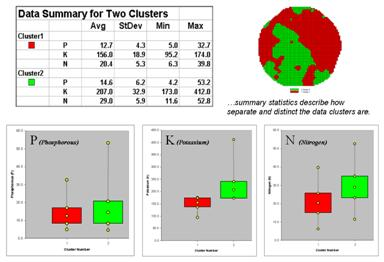Figure 3.  Clustering results can be roughly evaluated using basic statistics.

So how do know if the clustering results are acceptable?  Most statisticians would respond, “you can’t tell for sure.”  While there are some elaborate procedures focusing on the cluster assignments at the boundaries, the most frequently used benchmarks use standard statistical indices.

Figure 3 shows the performance table and box-and-whisker plots for the map containing two clusters.  The average, standard deviation, minimum and maximum values within each cluster are calculated.  Ideally the averages would be radically different and the standard deviations small—large difference between groups and small differences within groups.

Box-and-whisker plots enable us to visualize these differences.  The box is centered on the average (position) and extends above and below one standard deviation (width) with the whiskers drawn to the minimum and maximum values to provide a visual sense of the data range.  When the diagrams for the two clusters overlap, as they do for the phosphorous responses, it tells us that the clusters aren’t very distinct along this axis.   The separation between the boxes for the K and N axes suggests greater distinction between the clusters.  Given the results a practical PF’er would likely accept the classification results… and statisticians hopefully will accept in advance my apologies for such a conceptual and terse treatment of a complex topic.

Talk about the future of precision Ag… how about maps of things yet to come?  Sounds a bit far fetched but Spatial Data Mining is taking us in that direction.  For years non-spatial statistics has been predicting things by analyzing a sample set of data for a numerical relationship (equation) then applying the relationship to another set of data.  The drawbacks are that the non-approach doesn’t account for geographic relationships and the result is just a table of numbers addressing an entire field.

Extending predictive analysis to mapped data seems logical.  After all, maps are just organized sets of numbers.  And the GIS toolbox enables us to link the numerical and geographic distributions of the data.  The past several columns have discussed how the computer can “see” spatial data relationships— “descriptive techniques” for assessing map similarity, classification units and data zones.  The next logical step is to apply “predictive techniques” to generate extrapolative maps.

If fact, the first time I used prediction mapping was in 1991 to extend a test market project for a phone company.  The customer’s address was used to geo-code sales of a new product that enabled two numbers with distinctly different rings to be assigned to a single phone— one for the kids and one for the parents.  Like pushpins on a map, the pattern of sales throughout the city emerged with some areas doing very well, while in other areas sales were few and far between.

The demographic data for the city was analyzed to calculate a prediction equation between product sales and census block data.  The prediction equation derived from the test market sales in one city was applied to another city by evaluating exiting demographics to “solve the equation” for a predicted sales map.  In turn the predicted map was combined with a wire-exchange map to identify switching facilities that required upgrading before release of the product in the new city.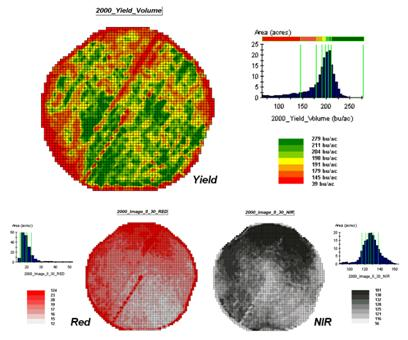Figure 1.  The corn yield map (top) identifies the pattern to predict; the red and near-infrared maps (bottom) are used to build the spatial relationship.

To illustrate the data mining procedure, the approach can be applied to the cornfield data that has been focus for the past several columns.  The top portion of figure 1 shows the yield pattern for the field varying from a low of 39 bushels per acre (red) to a high of 279 (green).  Corn yield, like “sales yield,” is termed the dependent map variable and identifies the phenomena we want to predict.

The independent map variables depicted in the bottom portion of the figure are used to uncover the spatial relationship— prediction equation.  In this instance, digital aerial imagery will be used to explain the corn yield patterns.  The map on the left indicates the relative reflectance of red light off the plant canopy while the map on the right shows the near-infrared response (a form of light just beyond what we can see).

While it is difficult for you to assess the subtle relationships between corn yield and the red and near-infrared images, the computer “sees” the relationship quantitatively.  Each grid location in the analysis frame has a value for each of the map layers— 3,287 values defining each geo-registered map covering the 189-acre field.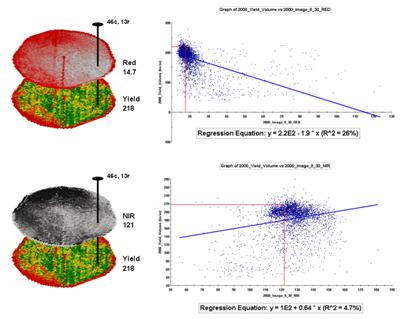Figure 2.  The joint conditions for the spectral response and corn yield maps are summarized in the scatter plots shown on the right.

For example, top portion of figure 2 identifies that the example location has a “joint” condition of red equals 14.7 counts and yield equals 218 bu/ac.  The red lines in the scatter plot on the right show the precise position of the pair of map values—X= 14.7 and Y= 218.  Similarly, the near-infrared and yield values for the same location are shown in the bottom portion of the figure.

In fact the set of “blue dots” in both of the scatter plots represents data pairs for each grid location.  The blue lines in the plots represent the prediction equations derived through regression analysis.  While the mathematics is a bit complex, the effect is to identify a line that “best fits the data”— just as many data points above as below the line.

In a sense, the line sort of identifies the average yield for each step along the X-axis (red and near-infrared responses respectively).  Come to think of it, wouldn’t that make a reasonable guess of the yield for each level of spectral response?  That’s how a regression prediction is used… a value for red (or near-infrared) in another field is entered and the equation for the line is used to predict corn yield.  Repeat for all of the locations in the field and you have a prediction map of yield from an aerial image… but alas, if it were only that simple and exacting.

A major problem is that the “r-squared” statistic for both of the prediction equations is fairly small (R^^2= 26% and 4.7% respectively) which suggests that the prediction lines do not fit the data very well.  One way to improve the predictive model might be to combine the information in both of the images.  The “Normalized Density Vegetation Index (NDVI)” does just that by calculating a new value that indicates relative plant vigor— NDVI=  ((NIR – Red) / (NIR + Red)).

Figure 3 shows the process for calculating NDVI for the sample grid location— ((121-14.7) / (121 + 14.7))= 106.3 / 135.7= .783.  The scatter plot on the right shows the yield versus NDVI plot and regression line for all of the field locations.  Note that the R^^2 value is a higher at 30% indicating that the combined index is a better predictor of yield.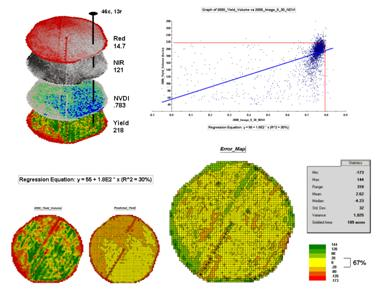Figure 3.  The red and NIR maps are combined for NDVI value that is a better predictor of yield.

The bottom portion of the figure evaluates the prediction equation’s performance over the field.  The two smaller maps show the actual yield (left) and predicted yield (right).  As you would expect the prediction map doesn’t contain the extreme high and low values actually measured.

However the larger map on the right calculates the error of the estimates by simply subtracting the actual measurement from the predicted value at each map location.  The error map suggests that overall the yield “guesses” aren’t too bad— average error is a 2.62 bu/ac over guess; 67% of the field is within 20 bu/ac.  Also note that most of the over estimating occurs along the edge of the field while most of the under estimating is scattered along curious NE-SW bands.

While evaluating a prediction equation on the data that generated it isn’t validation, the procedure provides at least some empirical verification of the technique.  It suggests a glimmer of hope that with some refinement the prediction model might be useful in predicting yield before harvest.  Next month we’ll investigate some of these refinement techniques and see what information can be gleamed by analyzing the error surface.

The last section described procedures for predictive analysis of mapped data.  While the underlying theory, concerns and considerations can easily consume a graduate class for a semester the procedure is quite simple.  The grid-based processing preconditions the maps so each location (grid cell) contains the appropriate data.  The “shishkebab” of numbers for each location within a stack of maps are analyzed for a prediction equation that summarizes the relationships.

In the example discussed in the last section, regression analysis was used to relate a map of NDVI (“normalized density vegetation index” derived from remote sensing imagery) to a map of corn yield for a farmer’s field.  Then the equation was used to derive a map of predicted yield based on the NDVI values and the results evaluated for how well the prediction equation performed.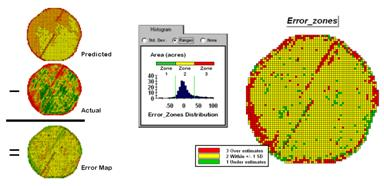Figure 1.  A field can be stratified based on prediction errors.

The left side of figure 1 shows the evaluation procedure.  Subtracting the actual yield values from the predicted ones for each map location derives an Error Map.  The previous discussions noted that the yield “guesses” weren’t too bad—average error of 2.62 bu/ac with 67% of the estimates within 20 bu/ac of the actual yield.  However, some locations were as far off as 144 bu/ac (over-guess) and –173 bu/ac (under-guess).

One way to improve the predictions is to stratify the data set by breaking it into groups of similar characteristics.  The idea is that set of prediction equations tailored to each stratum will result in better predictions than a single equation for an entire area.  The technique is commonly used in non-spatial statistics where a data set might be grouped by age, income, and/or education prior to analysis.  In spatial statistics additional factors for stratifying, such as neighboring conditions and/or proximity, can be used.

While there are several alternatives for stratifying, subdividing the error map will serve to illustrate the conceptual approach.  The histogram in the center of figure 1 shows the distribution of values on the Error Map.  The vertical bars identify the breakpoints at plus/minus one standard deviation and divide the map values into three strata—zone 1 of unusually high under-guesses (red), zone 2 of typical error (yellow) and zone 3 of unusually high over-guesses (green).  The map on the right of the figure locates the three strata throughout the field.

The rationale behind the stratification is that the whole-field prediction equation works fairly well for zone 2 but not so well for zones 1 and 3.  The assumption is that conditions within zone 1 makes the equation under estimate while conditions within zone 3 cause it to over estimate.  If the assumption holds one would expect a tailored equation for each zone would be better at predicting than an overall equation.  Figure 2 summarizes the results of deriving and applying a set of three prediction equations.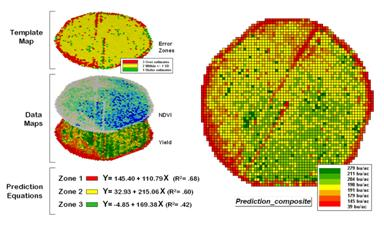Figure 2.  After stratification, prediction equations can be derived for each element.

The left side of the figure illustrates the procedure.  The Error Zones map is used as a template to identify the NDVI and Yield values used to calculate three separate prediction equations.  For each map location, the algorithm first checks the value on the Error Zones map then sends the data to the appropriate group for analysis.  Once the data has been grouped a regression equation is generated for each zone.  The “r-squared” statistic for all three equations (.68, .60, and .42 respectively) suggests that the equations fit the data fairly well and ought to be good predictors.  The right side of figure 2 shows the composite prediction map generated by applying the equations to the NDVI data respecting the zones identified on the template map.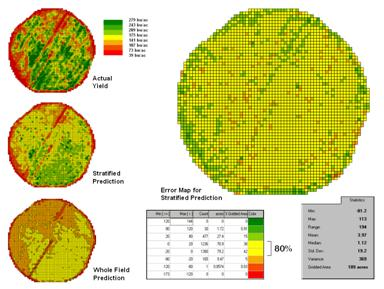Figure 3.  Stratified and whole-field predictions can be compared using statistical techniques.

The left side of figure 3 provides a visual comparison between the actual yield and predicted maps.  The “stratified prediction” shows detailed estimates that more closely align with the actual yield pattern than the “whole-field” derived prediction map.  The error map for the stratified prediction shows that eighty percent of the estimates are within +/- 20 bushels per acre.   The average error is only 4 bu/ac with maximum under and over-estimates of –81.2 and 113, respectively.  All in all, not bad guessing of yield based on a remote sensing shot of the field nearly a month before the field was harvested.

A couple of things should be noted from this example of spatial data mining.  First, that there is a myriad of other ways to stratify mapped data—1) Geographic Zones, such as proximity to the field edge; 2) Dependent Map Zones, such as areas of low, medium and high yield; 3) Data Zones, such as areas of similar soil nutrient levels; and 4) Correlated Map Zones, such as micro terrain features identifying small ridges and depressions.  The process of identifying useful and consistent stratification schemes is an emerging research frontier in the spatial sciences.

Second, the error map is key in evaluating and refining the prediction equations.  This point is particularly important if the equations are to be extended in space and time.  The technique of using the same data set to develop and evaluate the prediction equations isn’t always adequate.  The results need to be tried at other locations and dates to verify performance.  While spatial data mining methodology might be at hand, good science is imperative.

The bottom line is that maps are increasingly seen as organized sets of data that can be map-ematically analyzed for spatial relationships… we have only scratched the surface.  The applications of spatial analysis and data mining in production agriculture are in their infancy.  As the agricultural sciences embrace spatial technology, research will tailor the procedures for the unique data and situations facing farmers.

The Precision Farming Primer is intended to spark this revolution… the wildfire of change will come from the agronomists, soil scientists, crop consultants, equipment dealers, chemical distributors and innovative farmers.  The effect will be farmland management more in tune with the spatial patterns within fields.  The result will be improved management practices that increase productivity while reducing environmental problems.  While the trappings of precision agriculture might appear unfamiliar (and even uncomfortable) at the onset, the technology will soon become second nature like those of the previous “mechanical” and “green” revolutions that preceded it.

Grid-based map analysis involves two broad categories of operations—Spatial Statistics and Spatial Analysis.  Table 4-1 outlines some of the specific techniques within each category.  Spatial Statistics tools, like the ones presented in the previous discussions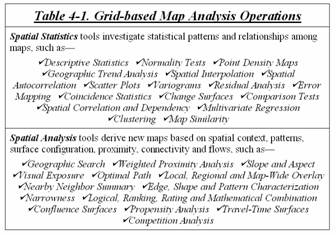on comparing maps, interpolation, data grouping and predictive models, respond to “numerical” relationships in mapped data.

Spatial Analysis tools, on the other hand, investigate “contextual” relationships, such as slope/aspect, buffers, effective proximity, optimal path, visual exposure and shape analysis.  Rather than statistical investigation of mapped data relationships, these techniques examine geographic patterns, vicinity characteristics and connectivity among features.

From this perspective, four classes of spatial analysis techniques can be identified that— reclassify map categories, overlay two or more maps, measure distance and connectivity, and characterize cartographic neighborhoods.

In the broadest sense, Reclassification operations merely repackage existing information on a single map.  Overlay operations, on the other hand, involve two or more maps and result in the delineation of new information.  Distance operations generate entirely new information by characterizing the relative positioning of map features.   Neighborhood operations summarize the conditions occurring in the general vicinity of a location.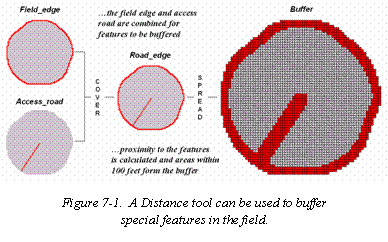Figure 7-1.  A Distance tool can be used to buffer
special features in the field.

While precision agriculture often involves spatial statistics in its applications, most other GIS applications focus on spatial analysis operations, particularly the “geo-query” capability to retrieve locations of map features stored in a database.

For example, a forester might want to identify forest parcels of Douglas fir on Cohasset soil within a county.  Or a real estate agent might query the residential listing for houses within a specified distant of a school and costing less than \$300,000.

_____________________

Author’s note:  An educational version of the MapCalc software by Red Hen Systems used in the case study provides “hands-on” exercises for the topics discussed in this Case Study series.   See www.redhensystems.com for more information and ordering; US\$21.95 for Student CD and US\$495.00 for Instructor CD with multi-seat license for a computer lab (prices subject to change).

______________